Next: Orbital energies Up: Keplerian orbits Previous: Kepler's third law

# Orbital parameters

For an elliptical orbit, the closest distance to the Sun--the so-called perihelion distance--is [see Equation (4.30)]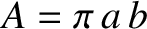(4.34)

This equation also holds for parabolic and hyperbolic orbits. Likewise, the furthest distance from the Sun--the so-called aphelion distance--is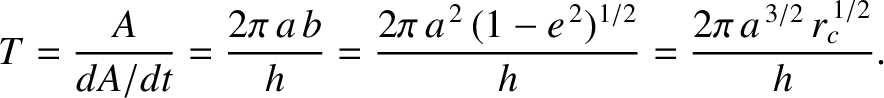(4.35)

It follows that, for an elliptical orbit, the major radius,, is simply the mean of the perihelion and aphelion distances,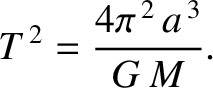(4.36)

The parameter(4.37)

is called the eccentricity and measures the deviation of the orbit from circularity. Thus,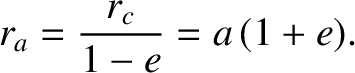corresponds to a circular orbit, whereas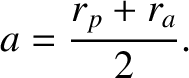corresponds to an infinitely elongated elliptical orbit. Note that the Sun is displaced a distancealong the major axis from the geometric center of the orbit. (See Section A.9 and Figure 4.3.)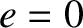As is easily demonstrated from the preceding analysis, Kepler laws of planetary motion can be written in the convenient form(4.38)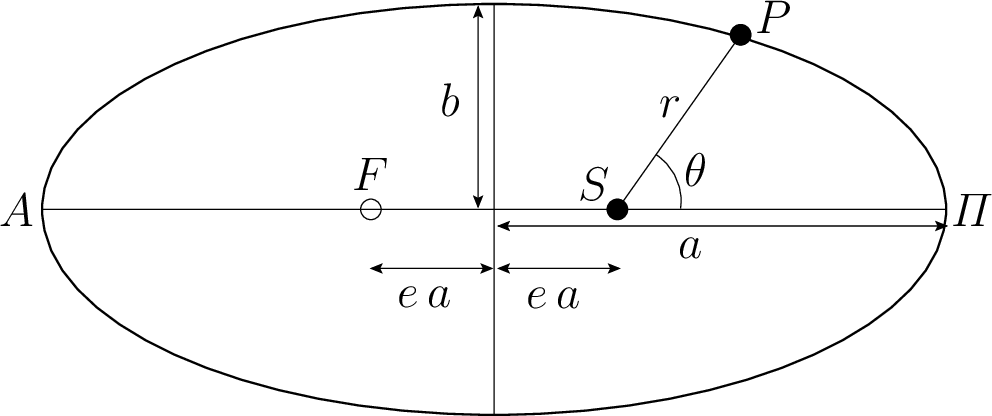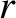(4.39) and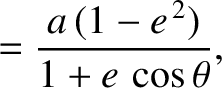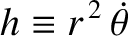(4.40)

whereis the mean orbital radius (i.e., the major radius),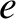the orbital eccentricity, and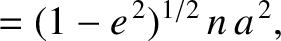the mean orbital angular velocity.Next: Orbital energies Up: Keplerian orbits Previous: Kepler's third law
Richard Fitzpatrick 2016-03-31